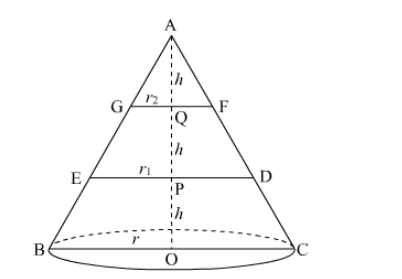# A right circular cone is divided into three parts by trisecting its height by two planes drawn parallel to the base.`
Question:

A right circular cone is divided into three parts by trisecting its height by two planes drawn parallel to the base. Show that the volume of the three portions starting from the top are in the ratio 1 : 7 : 19.

Solution:

Let ABC be a right circular cone of height 3h and base radius r. This cone is cut by two planes such that AQ = QP = PO = h.Since $\Delta \mathrm{ABO} \sim \Delta \mathrm{AEP}$ (AA Similarity)

$\therefore \frac{\mathrm{AO}}{\mathrm{AP}}=\frac{\mathrm{BO}}{\mathrm{EP}}$

$\Rightarrow \frac{3 h}{2 h}=\frac{r}{r_{1}}$

$\Rightarrow r_{1}=\frac{2 r}{3} \quad \ldots . .(1)$

Also, $\triangle \mathrm{ABO} \sim \Delta \mathrm{AGQ} \quad$ (AA Similarity)

$\therefore \frac{\mathrm{AO}}{\mathrm{AQ}}=\frac{\mathrm{BO}}{\mathrm{GQ}}$

$\Rightarrow \frac{3 h}{h}=\frac{r}{r_{2}}$

$\Rightarrow r_{2}=\frac{r}{3} \quad \ldots \ldots(2)$

Now,
Volume of cone AGF,

$V_{1}=\frac{1}{3} \pi r_{2}^{2} h$

$=\frac{1}{3} \pi\left(\frac{r}{3}\right)^{2} h \quad[$ From $(2)]$

$=\frac{1}{27} \pi r^{2} h$

Voulme of the frustum GFDE,

$V_{2}=\frac{1}{3} \pi\left(r_{1}^{2}+r_{2}^{2}+r_{1} r_{2}\right) h$

$=\frac{1}{3} \pi\left(\frac{4 r^{2}}{9}+\frac{r^{2}}{9}+\frac{2 r^{2}}{9}\right) h \quad[$ From $(1)$ and $(2)]$

$=\frac{7}{27} \pi r^{2} h$

Voulme of the frustum EDCB,

$V_{3}=\frac{1}{3} \pi\left(r^{2}+r_{1}^{2}+r_{1} r\right) h$

$=\frac{1}{3} \pi\left(r^{2}+\frac{4 r^{2}}{9}+\frac{2 r^{2}}{3}\right) h \quad[$ From $(1)$ and $(2)]$

$=\frac{19}{27} \pi r^{2} h$

$\therefore$ Required ratio $=V_{1}: V_{2}: V_{3}=\frac{1}{27} \pi r^{2} h: \frac{7}{27} \pi r^{2} h: \frac{19}{27} \pi r^{2} h=1: 7: 19$